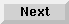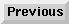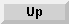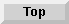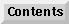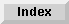7 Subprograms

## Parameters

Values are passed between a subprogram and the calling program segment using parameter lists. There are two kinds of parameters--formal and pass. Formal parameters are used to define the subprogram. Pass parameters are used to pass values from the calling program segment to the subprogram. Each pass parameter must correspond to a formal parameter.

The formal parameter list is used to define the subprogram variables and relate them to calling program variables. In addition, the parameter list includes non-subscripted numeric and string variable names, array identifiers (an array name followed by (*) specifies use of the entire array), User Defined Types(see section , User defined Types, on page 93) and file numbers (see page 195 ). Parameters must be separated by commas, and the parameter list must be enclosed in parentheses.

Numeric types REAL, SHORT, INTEGER and DINTEGER can be declared in a formal parameter list by placing the keyword before either a parameter or group of parameters. For example:

```10   SUB X(A,B\$,INTEGER C(*),D,SHORT E,F,#3,G)
```
The array C and simple variable D are declared as integer precision; E, F and G are short precision; and A is real precision. The #3 parameter refers to data file number 3.

NOTE: Instead of declaring a formal parameter to be a REAL, SHORT, INTEGER or DINTEGER, you could declare it as NUMERIC. Then it will be one of the passed type, i.e. if passed an INTEGER, it returns an INTEGER, if passed a REAL, it returns a REAL.

When calling a subprogram or function with a STRUCT argument, you can pass an object either anonymously or specify a type name.

CALL Sub(STRUCT A)

. . .

SUB Sub(A {AS|:} TypeName)

SUB Sub(STRUCT A)

Like regular variables, types can either be passed by value or by reference. When passed by value, a copy is passed to the subroutine.

For example:

```   TYPE Type
INTEGER I
END TYPE
!
DIM Inst:Type
Inst.I=0
CALL Sub(Inst)
PRINT Inst.I
CALL Sub((Inst))
PRINT Inst.I
STOP
!
SUB Sub(STRUCT A)
A.I=A.I+1
SUBEND
```
A STRUCT can be passed to a subprogram or function in two manners:

Instances can be passed to a SUBroutine either anonymous or with an associated type.

• When no type is specified in the SUBprogram or function definition, the struct is passed "anonymously" as an argument. Any type is valid and no validation is performed on the argument. RTTI (TYPEOF\$, IS A) can be used to operate on it.

• When a type is specified in the SUBprogram or function definition, only variables of the given (or derived) type are accepted, else a runtime error 8 is issued. The type must have been exported previously.

For example:

```CALL X(STRUCT X)
SUB X(STRUCT Any)             ! Anonymous

CALL Y(STRUCT X)
SUB Y(STRUCT Known:Tknown)    ! Passed value must be of type Tknown
! or derived from it. Tknown must have
! been definined previously
```

The pass parameter list used in calling the subprogram can include numeric and string variable names, array elements and identifiers, numeric and string expressions, user defined types and data file numbers. The pass parameter list must also be enclosed in parentheses.

Parameters must be separated by commas. All arrays in the pass parameter list must be defined within the calling program segment.

When a subprogram is called, each formal parameter corresponds to, and is assigned, the value of the pass parameter which is in the corresponding position in the pass parameter list. The parameter lists must have the same number of parameters, and the parameters must match in type--numeric precision or string, simple or array, or file. Notice that numeric types must match in precision--real, integer, or short.

Notice the correspondence between pass and formal parameters in the next example:

```20   INTEGER C(2,2),D(2,2)
30   CALL X(A,B\$,C(*),(D(1,2)),3,E+F,#6,(G))
.
.
.
70   CALL X(5,(C\$[1,12]),D(*),4,(X(4,3)),(A),#2,E*3)
.
.
.
120  SUB X(X,Y\$,INTEGER Z(*),SHORT K,L,M,#9,N)
```
Parameters are passed either by reference or by value. When a parameter is passed by reference, the corresponding formal parameter shares the same memory area with the pass parameter. Thus, changing the value of the variable in the subprogram also changes the value of the variable in the calling program. Arrays are always passed by reference.

When a parameter is passed by value, the variable defined by the corresponding formal parameter is assigned the value of the pass parameter and given temporary storage space in memory. Numeric and string expressions are necessarily passed by value. Enclosing a pass parameter in parentheses causes it to be considered an expression and thus passed by value, rather than by reference. Passing by value prevents the value of a calling program variable from being changed within a subprogram.

In the following example, all parameters in line 200 are passed by value, while those in line 250 are passed by reference:

```200   CALL Active(Y+3,(X(2,4)),(X(1,4)),.5,(Y),(Line\$[5,8]))
.
.
.
250   CALL Active(Y,Data(2,4),X(1,4),A,Z,Line\$)
.
.
.
290   SUB Active(A,B,C,D,E,F\$)
```
Any parameters passed by value are converted, if necessary, to the numeric type of the corresponding parameter in the formal parameter list. For example, say that PI is passed by value to an INTEGER formal parameter. Its value would be rounded to 3 when the subprogram is called.

Those passed by reference must match exactly, otherwise Error 8 occurs. No conversion is made.

For instance:

```10   DIM C(3,3),D(3,3)
20   CALL X(A,B\$,C(*),D(1,2),3,E,#5,G)
30   END
40   SUB X(X,Y\$,INTEGER Z(*),SHORT K,L,M,#3,N)
50   SUBEND
60   END

RUN
ERROR 8 IN LINE 20
```
NUMERIC is used in parameter passing to subroutines and functions. NUMERIC will bypass type checking for parameters and will match any numeric data type.

Example program:

```REAL R
INTEGER I

CALL Val(A\$,R)
CALL Val(A\$,I)

SUB Val(A\$, NUMERIC V)
ON ERROR GOTO E
V=VAL(A\$)
SUBEXIT
E: V=0
SUBEND
```

Eloquence Language Manual - 19 DEC 2002